Web Programming TutorialsLearn about string and integer handling changes in PHP 7

# Learn about string and integer handling changes in PHP 7In this chapter, we are going to discuss the various changes made to String and Integer handling in PHP 7 which are backward incompatible to PHP 5.x and other older versions of PHP.

Backward incompatible changes to Integer in PHP 7
The following are the backward incompatible changes made to Integer in PHP 7 which differ in output when compared to PHP 5 or other older versions of PHP.

• Parsing error – Invalid octal literals: In PHP 5.x, when we assign an extra digit to an octal literal, it truncates that extra digit. E.g., 0148 will be truncated to 014, etc. However in PHP 7.x, if we assign an extra digit to an octal literal, then it will throw a parse error after considering it as an invalid octal literal at runtime. Let’s understand this with the help of the following example.
```<html>
<title><?php echo "Welcome to PHP-7 Tutorials";?></title>
<body>
<?php
\$a = 0124; // octal number (equivalent to 84 decimal)
print("Octal equivalent number: "+\$a);
\$b = 01248; // octal number (equivalent to 84 decimal in PHP 5 but not in PHP 7)
print("<br>Octal equivalent number: "+\$b);
?>
</body>
</html>```

Output
When we execute the above program on the web server, then we will observe the following parse error as shown below.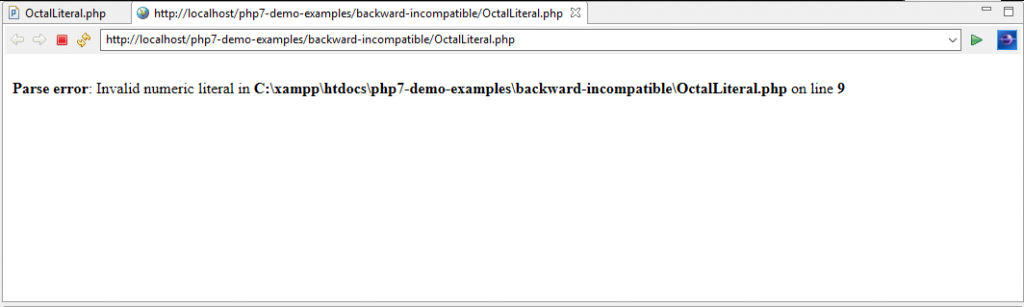• ArithmeticError – Negative bitshifts: In PHP 7, when we do bitwise shifts by negative numbers, then it will throw an ArithmeticError. However in PHP 5.x, this was not the case. Let’s understand this with the help of the following example.
```<html>
<title><?php echo "Welcome to PHP-7 Tutorials";?></title>
<body>
<?php
var_dump(12 >> -1);
?>
?>
</body>
</html>```

Output
When we execute the above PHP 7 program on the web server, we see the following ArithmeticError as shown below.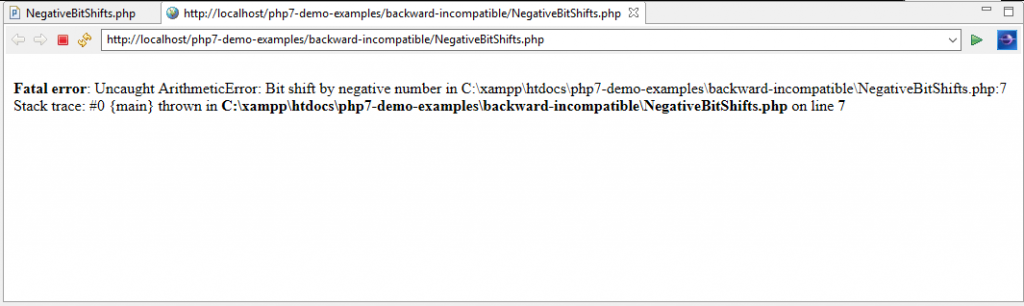• Out of range bitshifts in PHP 7: In PHP 7, when we do the bitwise shifting (in both directions i.e. left or right) beyond the bit width of an integer value, then it will always result a zero value. However in the older version versions of PHP (PHP 5.x, etc.), the behaviour of such bitwise shifting was dependant on the system architecture. Let’s understand this with the help of the following example.
```<html>
<title><?php echo "Welcome to PHP-7 Tutorials";?></title>
<body>
<?php
var_dump(1 >> 3);
?>
</body>
</html>```

Output
When we execute the above PHP 7 program on the web server, we see the following zero result on bitwise shifting of the out of range integer number (here 1) as shown below.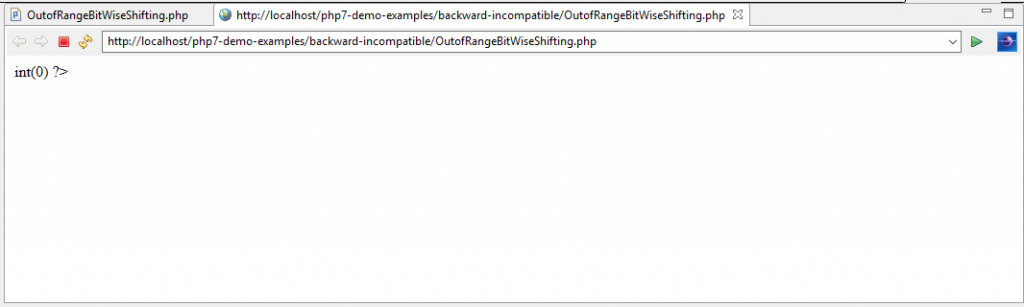• Changes to Division by zero in PHP 7: In PHP 5.x versions, when we attempt to do division (/) or modulus (%) of a number by zero (0), then the PHP 5 parser issues an E_WARNING and returns a Boolean false value. However in PHP 7 this changes. Here, the divide operator returns a float value which could be +INF, -INF, or NAN, as specified by IEEE 754 and the E_WARNING has been removed.

PHP 7 parser will throw a DivisionByZeroError exception when it encounters such scenario of division (/) or modulus (%) of a number by zero (0). Let’s look at an example.

```<html>
<title><?php echo "Welcome to PHP-7 Tutorials";?></title>
<body>
<?php
var_dump(89/0);
var_dump(0/0);
var_dump(0%0);
var_dump(89%0);
?>
</body>
</html>```

Output
When we execute the above PHP 7 program on the web server then we will observe the following Division by zero exception as float (INF), float (NAN) as shown below. In case of modulus by zero operation, PHP 7 will throw Fatal Error.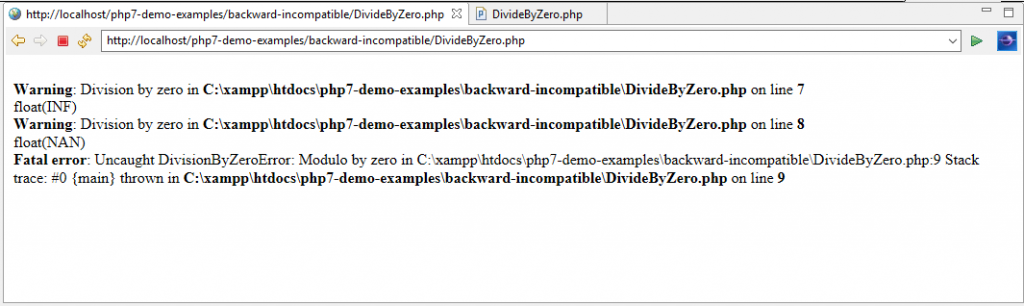Backward incompatible changes made to String in PHP 7
The following are the backward incompatible changes made to string in PHP 7 which differ in output when compared to PHP 5 or other older versions of PHP.

• Hexadecimal strings are not numeric in PHP 7: Strings that contain hexadecimal numbers are not considered as number by the PHP 7 parser, which were originally considered as number within the earlier versions of PHP. Let’s understand this with the help of the following example.
```<html>
<title><?php echo "Welcome to PHP-7 Tutorials";?></title>
<body>
<?php
var_dump("0x124" == "292");
print("<br />");
var_dump(is_numeric("0x124"));
print("<br />");
var_dump("0xd" + "0x3");
print("<br />");
var_dump(substr("PHP", "0x2"));
?>
</body>
</html>```

Output
When we execute the above PHP 7 program on the web server, we see that the hexadecimal string is no longer considered as a numeric value as shown below.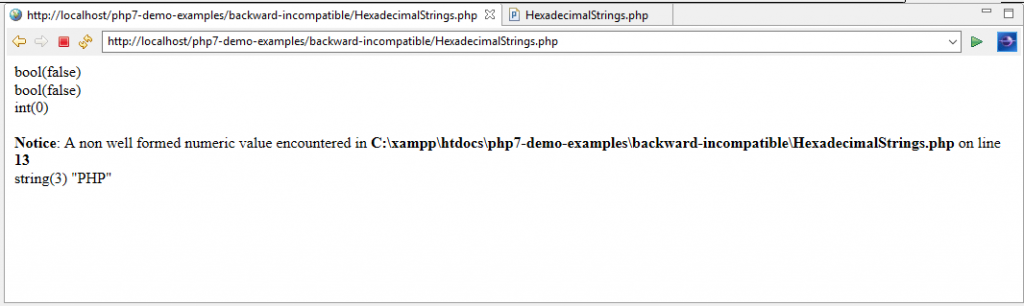• The function filter_var () in PHP 7: This function is used to serve the following purposes.
1. It is used to check whether a string contains hexadecimal numbers or not.
2. It is used to convert a string of that type into an integer value.
Let’s understand this with the help of the following example.
```<html>
<title><?php echo "Welcome to PHP-7 Tutorials";?></title>
<body>
<?php
\$strVal = "0xeacf";
\$intVal = filter_var(\$strVal, FILTER_VALIDATE_INT, FILTER_FLAG_ALLOW_HEX);
// To check that a string contains a hexadecimal number or not.
if (false === \$intVal) {
throw new Exception("Invalid integer!");
}
// To convert a string of that type into an integer value.
var_dump(\$intVal); // int(60111)
?>
</body>
</html>```

Output
When we execute the above PHP 7 program on the web server, we see the conversion of string of that type into an integer value as shown below.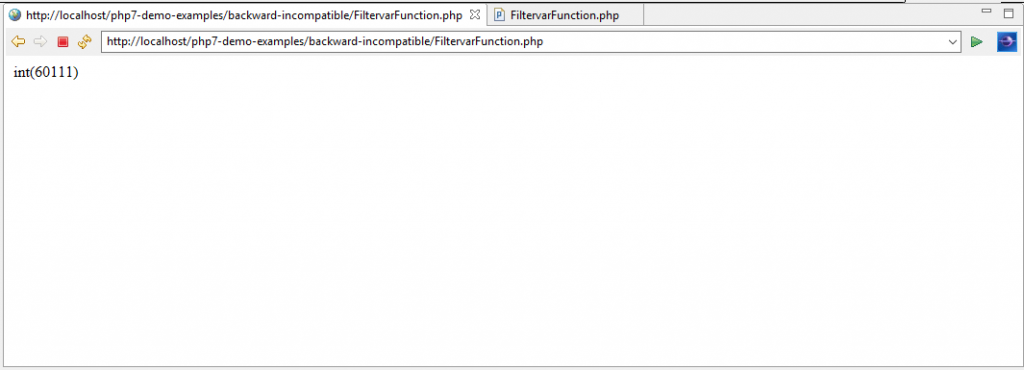• \u{ may cause errors in PHP 7: PHP 7 has added a new Unicode codepoint escape syntax due to which strings that contain a literal \u{ and followed by an invalid sequence will result in a fatal error. In PHP 7, it can be mitigated by escaping the leading backslash.
Source Code for  string and integer handling

Conclusion
In this chapter, we discussed about the various changes to String and Integer handling in PHP 7 along with examples.

### Why Is Design Thinking Important In Digital Transformation?

21,501Fans
4,210Followers
104,000Subscribers

### Why Is Design Thinking Important In Digital Transformation?

- Advertisement -